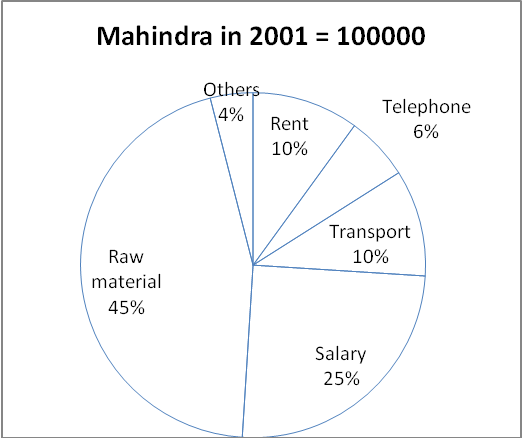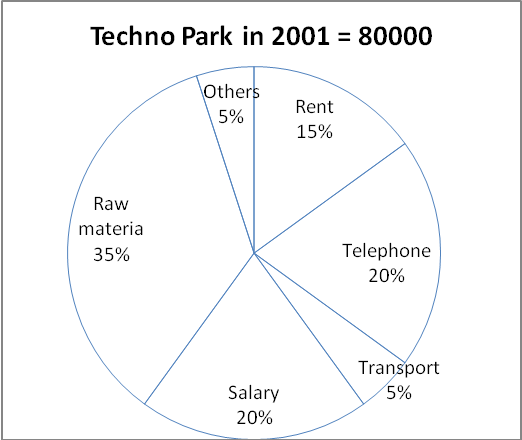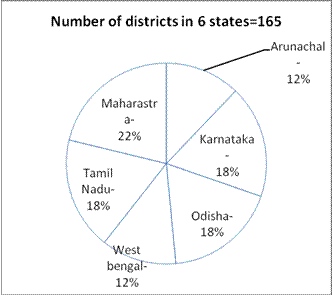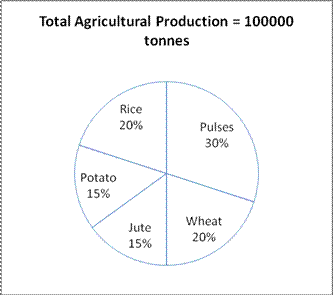# Data Interpretation-Pie Chart

D.1-5)The following pie charts show the break-up of the annual expenditures of two private sector companies:Q.1) The amount spent on transport by Techno Park is what percentage of the amount spent by Mahindra on transport?

(a) 30%

(b) 35%

(c) 40%

(d) 45%

(e) None of these

c

Amount spent by Technopark on transport = 5% of Rs. 80000 = Rs. 4000

Amount spent by Mahindra on transport = 10% of Rs. 100000 = Rs. 10000

= (4000 × 100)/10000= 40%

Q.2) The expenditure on raw material for both companies taken together, is what fraction of the total expenditure in 2001?

(a) 0.2

(b) 0.25

(c) 0.3

(d) 0.4

(e) None of these

d

Expenditure on raw material in both years=(45 + 35)/200

=75/200

= 0.4

Q.3) Both Mahindra and Techno Park spend the same amount on which category?

(a) Rent

(b) Transport

(c) Salaries

(d) Others

(e) None of these

d

Mahindra in 2001=100000,

telephone=100000×6/100=6000

Transport=100000×10/100=10000

Salary=100000×25/100=25000

Raw material=100000×45/100=450000

others=100000×4/100=4000

rent=100000×10/100=100000

Techno park in 2001=80000

Telephone=80000×20/100=16000

Transport=80000×5/100=4000

Salary=80000×20/100=16000

Raw material=80000×35/100=28000

Others=80000×5/100=4000

Rent=80000×15/100=12000

Therefore, Others for both the companies = Rs. 4000

Q.4) For which category the difference in expenditure is maximum?

(a) Telephone

(b) Transport

(c) Salaries

(d) Raw Material

(e) None of these

d

Mahindra in 2001=100000,

telephone=100000×6/100=6000

Transport=100000×10/100=10000

Salary=100000×25/100=25000

Raw material=100000×45/100=450000

others=100000×4/100=4000

rent=100000×10/100=100000

Techno park in 2001=80000

Telephone=80000×20/100=16000

Transport=80000×5/100=4000

Salary=80000×20/100=16000

Raw material=80000×35/100=28000

Others=80000×5/100=4000

Rent=80000×15/100=12000

For the category, raw material the difference is maximum

= 45000 – 28000 = Rs. 17000.

Q.5) The amount spent on salaries by Techno Park is what percentage more than the amount spent by Mahindra on rent?

(a) 50%

(b) 65%

(c) 60%

(d) 45%

(e) None of these

c

Amount spent by Technopark on salaries = 20% of Rs. 80000 = Rs. 16000

Amount spent by Mahindra on rent = 10% of Rs. 100000 = Rs. 10000

Required % = 6000/10000× 100=60% More

D.6-10)The following pie chart 1 shows number of districts in 6 states in India. And the chart 2 shows total agriculture products. By the two charts find the correct answer for the following questions.Q.6) If the 30% of Pulses supply to all the 6 states given in the chart equally and the states supply those pulses to all the district equally, then how much pulses is getting by each district in Odisha?

a) 120 tonnes

b) 70 tonnes

c) 50 tonnes

d) 65 tonnes

e) 45 tonnes

c

Total production = 100000 tonnes

Pulses = 30% = 30000 tonnes

30% of pulses = 9000 tonnes

Each states get = 9000/6= 1500

odisa districs=18/100×165=30

Odisha districts are 30, so each district get 1500/30= 50 tonnes

Q.7) If cultivation of Pulses increase by 10% and the total cultivation are remaining same.  The increase of Pulses cultivation affects the cultivation of Potato. Then what percent of potato cultivation is decrease after the increase of Pulses cultivation?

a) 10%

b) 20%

c) 15%

d) 25%

e) 30%

b

Pulses = 30% = 30000 tonnes

10% increase = 3000 tonnes

Potato = 15% = 15000 tonnes

Decrease = 3000 tonnes

% of decrease = 3000/15000× 100 = 20%

Q.8) Potato, Jute and Rice are exported to another country and the remaining is supply to all districts in India equally. Assume the country has 700 districts approximately. Then how much will west Bengal get?

a) 2100 tonnes

b) 3300 tonnes

c) 1429 tonnes

d) 2700 tonnes

e) 1800 tonnes

c

Total production of Potato Jute and rice = 15 + 15 + 20 = 50%

Which is 50000 ton.

Total district in the country = 700

Districts is West Bengal = 20

Share of West Bengal = 50000/700× 20 = 1428.5~1429 tonnes.

Q.9) Because of drought crops cultivation decreases on Pulses by 10%, wheat by 20%, Jute by 30%, Potato by 20% and Rice by 10%. What is the total effect after drought?

a) 15.5% decrease

b) 25.5% decrease

c) 18% decrease

d) 16.5% decrease

e) 19% decrease

d

Pulses = 100000 × 30/100×90/100= 27000

Wheat = 100000 × 20/100×80/100= 16000

Jute = 100000 × 15/100×70/100= 10500

Potato = 100000 × 15/100×80/100= 12000

Rice = 100000 × 20/100×90/100= 18000

Total = 27000 + 16000 + 10500 + 12000 + 18000 = 83500.

100000 – 83500 = 16500.

% of Decrease = 16500/100000× 100 = 16.5%

Q.10) If the 35% of total cultivation are allocated to South India, then How much will Tamil Nadu get, (Assume South India has 200 districts)?

a) 5250 tonnes

b) 5500 tonnes

c) 5000 tonnes

d) 3500 tonnes

e) 4500 tonnes